# Arrays in C Programming with Examples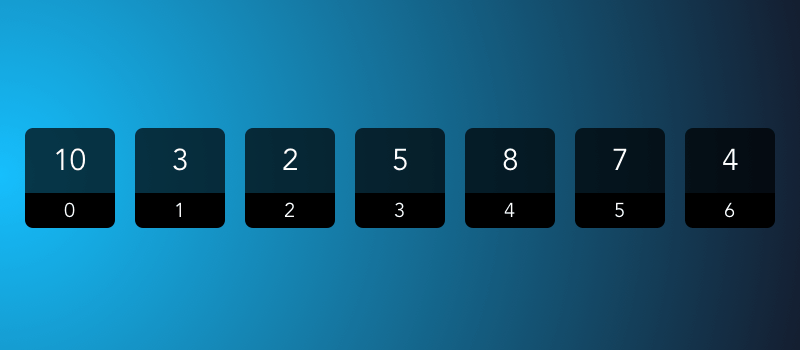Arrays in C allow you to store multiple items of the same data type, such as a list of integers. Arrays form the basis for many data structures and allow you to build advanced programs.

In this article, we are going to discuss what an array is and how you can use them, along with examples. We will also see the concept of “strings”, which is closely related to the topic of arrays.

Contents

## What is an array in C and why should you use them?

In C, an array is a way to store a fixed number of items of the same data type under a single name. Each data item of the array can be accessed by using a number called an “index” or “subscript”.

You might think, why do we need arrays to store multiple data types, when you can just declare normal variables? Let us take an example — suppose, you have been asked to write a program that takes in the temperature for the last 90 days and perform some processing on that data.

With enough time and patience, you can declare 90 integer variables (such as a1, a2, a3 and so on) to store this information. However, processing the information in these 90 variables is nearly impossible. What if you wanted to know the average temperature between a range of days (such as the 10th and 25th day), where this range would be given by the user?

While you can’t do this with normal variables, you can use arrays to make such a program. You can store all the temperature values under a single variable like a, and then extract any set of values from it by using the index.

## Declaring and using an array in C

To declare an array, you simply need to specify the data type of the array elements, the variable name and the array size. For example, if you want to declare an integer array with four elements, you’d use:

int a;


This statement allocates a contiguous block of memory for four integers and initializes all the values to 0. This is how it is laid out in memory: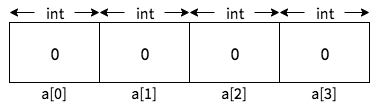Array indexes start from zero and end with (array size – 1). So for the above array, you can use the first element with a, second element with a, third element with a and fourth (last) element with a.

You can use the indexes to set or get specific values from the array. Here are a few examples:

a = 10;
a = 20;
a = a / a; // a will be set to 20/10 = 2
a = a - 2;    // a will be set to 20-2 = 18


After these changes, here is how the array will look like in memory:Apart from the square brackets to indicate the index, array elements behave like normal variables. So, for example, you can print them by using:

printf("%d %d %d %d\n", a, a, a, a);


You can see the full program in action below, or download it here.

One important thing to note is that C does not enforce any array bounds checks, and accessing elements outside the maximum index will lead to “undefined behaviour”. So, in the above example, trying to get or set a value like a can cause your program to crash or behave abnormally.

## Alternative ways to initialize arrays

Previously, we’ve seen how to declare an array and set its elements. However, if you know the elements of the array, then there is an easier way to declare the array. For example, you want to declare an integer array with the values 10, 20, 30, 40, you can use the “initializer list” syntax:

int a = {10, 20, 30, 40};


This statement will automatically create an array of size 4, and initialize a to 10, a to 20 and so on. To confirm that this works, you can print the variables, just like we did in our previous program.

You can also make an array that is bigger than the initializer list, like so:

int a = {10, 20, 30, 40};


In this case, the rest of the elements are initialized with zero. In our above example, elements from a to a will be initialized, whereas a and a will be set to zero. Again, you can easily verify this by writing a program:

You can also skip the array size when using initializer lists, like in the example below:

int a[] = {10, 20, 30};


The C compiler automatically guesses the size of the array from the size of the initializer list. So, the above example is the same as writing int a = {10, 20, 30};

However, you cannot skip both the size and the initializer list, and write int a[];. Arrays in C are of a fixed size, and so their size must be known when you create the array. Therefore, if you skip both of them, C cannot create the array, and this will lead to a compile-time error.

## Looping/iterating over an array in C

Previously, we have learnt how you can use the individual elements of an array. Since the array indexes are integers starting from 0 to (array size – 1), you can use loops to visit all the elements of the array. This process of visiting all the elements of an array is also called “traversing an array”.

Here is a very simple example. It initializes an array and prints each array element and the index:

In the for loop, the value of i starts with 0 (because we set i = 0) and ends at 3 (because the loop continues till i < 4). Inside the for loop, we print the value of a[i]. So, in the first iteration, we print the value of a. In the second iteration, we print a. In this way, we print all the elements of the array.

## Array program examples in C

Now that we know the basics of an array, we will look at some basic programs that use arrays in C.

### Reading user-entered numbers into an array

Let us begin with a simple program that reads five numbers into an array, and then prints them out. Here is the source code for the program, and you can download it here. (In this coding playground, you can change the input numbers via the “Input” tab.)

We begin by initializing an array of five elements and, a variable i which we’ll use for the for loop.

In the first loop, we read numbers from the user and set it in the a[i] element. The elements of an array simply behave like regular variables. If you had an int variable named p and wanted to set the value of p from user input, you’d use scanf("%d", &p). Similarly, here, to set the value of a[i] from user input, we used scanf("%d", &a[i]).

The second loop simply prints the variables one by one using printf().

### Linear search in an array

Sometimes, you may need to search for an element in an array. For example, given an array {10, 20, 30, 40}, you may want to know if 30 is present in the array.

Linear search is a simple technique to search for an element. We iterate over every element of the array to check if it matches with the number we’re looking for.

Here is the source code for the program, and you can download it here.

In this program, we have declared an array a, the loop variable i, the element to search for search. We have also declared another variable pos, which keeps track of the array index where we found the element we were searching. We’ve initialized pos to -1; the reason for doing so will become clear later on.

Next, we read the elements of the array as well as the number to search for.

In the final loop, we’ve implemented the linear search logic. We iterate through each element of the array, and check if a[i] is equal to the value of search. If it is equal, we set the pos variable to the current index i, since we found the element at index i. Then, we break out of the loop, since we’ve found our element and we don’t need to process any further.

Then, we compare the value of pos. The minimum value of an array index can be 0. So, if we find the value of pos to be -1, this means there was no match and we’ll print a message like “30 was not found”. Otherwise, we’ll print a message such as “30 was found at position 2”.

## Two-dimensional (2D) arrays in C

So far, we’ve looked at arrays where the element stores a simple data type like int. These arrays are sometimes called one-dimensional (1D) arrays.

Just as int or float are data types, an array is also a data type. Therefore, you can build an array who’s individual elements are 1D arrays. These kinds of arrays are called two-dimensional (2D) arrays.

To declare a 2D array, you need:

• the basic data type
• the variable name
• the size of the 1D arrays
• the number of 1D arrays, which combined together make up the 2D array.

Here is how you can declare a 2D array:

int a;


This statement allocates a contiguous block of memory. For our example, two 1D arrays combine together to form a 2D array, as you can see in the diagram below. Each 1D array can hold four integers. Also, just like 1D arrays, all the elements of the array are initialized to zero.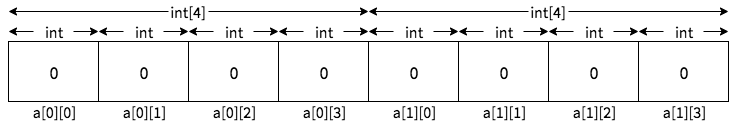Now, let us see how we can access the elements of a 2D array. Since a 2D array consists of multiple 1D arrays, you need two indexes. This is because, you need to specify the index of the 1D array, and the specific element inside that 1D array.

So, if you want to access the first 1D array’s second element, you should use a. Similarly, to access the second 1D array’s third element, you should use a and so on.

A very common approach is to visualize 2D arrays like a table, where the first index is the row number and the second index is the column number. So, you can represent the above array as: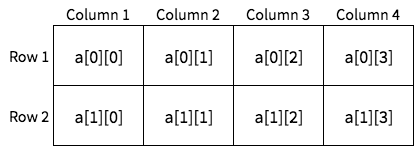This allows us to talk about 2D arrays in a much easier way. To use the element at the first row, third column, you can use a; to use the element at the second row, second column, use a and so on.

## Initializing, using and looping over 2D arrays

Apart from using two indexes, using 2D arrays is the same as using 1D arrays. A basic example of using the arrays is as follows:

int a;

a = 10;
a = a * 10;      // a will be set to 100
a = a / 5;       // a will be set to 20
a = a + a; // a will be set to 120


If you know the elements beforehand, you can also use the initializer list syntax that we discussed previously. For example, if you want to make the following array: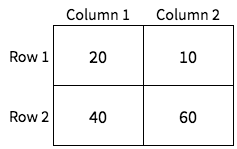You can write it as follows:

int a = {20, 10, 40, 60};


For easier understanding, you can also group the elements of the initializer list by using braces. For example, to initialize a 2D array with two rows and three columns, you can write:

int a = {{10, 20, 30}, {40, 50, 60}};


You can also loop over, print, or ask for input using scanf() as you would with 1D arrays. Here is an example of initializing an array, and then using for loops to print the elements. You can download the code here.

In the above program, we have used nested for loops to print the elements of the array. This is because a 2D array has two indexes, which means that the first and second index has to be changed individually. Since the printf() call uses a[i][j], so, the outer loop with variable i changes the first index, i.e. the row number. The inner loop with variable j changes the column number.

To understand this better, let us step through the loops. The outer loop starts with i = 0, and we get to the inner loop. The inner loop starts with j = 0 and ends at j = 2 (since j < 3), so we print a, a and a. After this, the inner loop value increases to 1, and similarly, we print a, a and a.

In this way, we print all the elements of the 2D array.

## 2D array program examples in C

In this section, we’re going to look at some basic programs involving 2D arrays in C. Since 2D arrays can be visualized in the form of a table or matrix, all of our examples will revolve around the concept of using them for matrix operations.

### Reading user-entered numbers into a 2D array

Before we look at more complex examples, let us first look at how to read numbers from the user into an array. Here’s the source code of the program, and you can download it here.

In the program, we begin by initializing an array of 10 rows and 10 columns, along with loop variables as well as variables that store the number of rows and columns requested by the user. Next, we ask the user to enter the number of rows and columns, and then we check if the number of rows and columns will fit into the 2D array. If it doesn’t fit, we print an error and exit the program.

Then, in the first nested for loop, we ask the user to enter the elements with scanf(). When printing the message “Enter row X, column Y”, we have used i + 1 and j + 1 ; this is because row/column numbers start from 1, but array indexes start from 0.

In the second nested for loop, we simply print the elements as we’ve seen in our previous example.

### Finding the transpose of a matrix

When you “transpose” a matrix, you take every element at the ith row and jth column of the matrix, and put it in the jth row and ith column. The formula for transpose is as follows:As you can see in this example, the size of a matrix also changes when you “transpose” it. A matrix of sizechanges to a matrix of size.

We can translate the above program into code quite easily. Suppose, the input array is a and the result array is b. Elements in a[i][j] will go into a b[j][i]. Here’s the source code for the program, and you can download it here:

Just like our previous example, we declare the arrays are a and b respectively and have 10 rows and 10 columns to hold the input and result matrix. Next, we ask the user to enter the rows and columns. If it exceeds the size of the array, we print an error and exit.

Then, we ask the user to enter the numbers one by one and print the input matrix. After that, we iterate through the matrix using a nested for loop, and implement the logic where a[i][j] goes to b[j][i].

As we discussed, the matrix size changes when you transpose it. In all the other loops, we were comparing i < rows and j < cols, but now since the size has changed, we should use i < cols and j < rows. So, in the last loop, we use this idea and print all the elements of the matrix.

In order to add two matrices, both must have the same number of rows and columns. The process of adding two matrices involves taking numbers from the same position of the matrix, adding them, and putting the result in the same position. The formula for matrix addition is as follows:So, if we have the two input matrices a and b and the result matrix c, then you can use c[i][j] = a[i][j] + b[i][j] in a loop to add the matrices. Here’s the source code for the program, and you can download it here:

In this program, we have two input matrices a and b and one result matrix c, and each of them are of 10×10 size. First, we ask the user for the size of the matrices, and if they’re bigger than the arrays, we print an error and exit.

Otherwise, we ask the user to enter the values in the input matrix. Then, using a nested for loop, we implement the process of matrix addition, c[i][j] = a[i][j] + b[i][j]. Finally, we print all the three matrices.

### Multiplying two matrices

Let us assume we have two matrices. One is of sizeand the other one is. These matrices can be only multiplied if. After multiplying, the result matrix will have a size of.

Now, to calculate the result in the ith row and jth column of the result matrix, take all the elements of the ith row of the first matrix, and multiply it with the corresponding value in the jth column. Then, take all these products and fill the sum in the result matrix. To see what we mean, let us take an example of how you can multiply two 2×2 matrices:The program that implements this logic is below. You can download it here.

In the program, we first declare 10×10 input and result matrices, along with some loop variables. Just like our previous programs, we ask the user for the sizes of the two matrices, and check if they are bigger than the 10×10 size. In addition, we check if the number of columns in the first matrix equals the number of rows in the second matrix. If any of these conditions fail, we print an error and exit the program.

Then, we ask the user to enter the numbers for both matrices. Once we’ve read all the numbers, we calculate the product with a nested loop. The two outer loops with variables i and j are used to move across the different elements of the result matrix. Initially, we set c[i][j] = 0. Now, we need to pick all the elements from the ith row of the first matrix and jth column from the second matrix. So, we use another loop with the variable k that helps us with this and multiplies and adds all the elements.

Finally, we display the input and result matrices.

## Multidimensional arrays in C

In the previous sections, we have seen 1D and 2D arrays. Any array with more than one dimension is a multidimensional array, and the concept can be extended to any number of dimensions, with the basics staying the same.

For example, a three dimensional (3D) array is a combination of multiple 2D arrays, and you can initialize a three dimensional array by using something like:

int a;


This statement creates a 3D array which is a combination of three 2×2 2D arrays. As with all arrays in C, memory allocation is contiguous and all the elements is initialized to zero. You can think of this array as follows: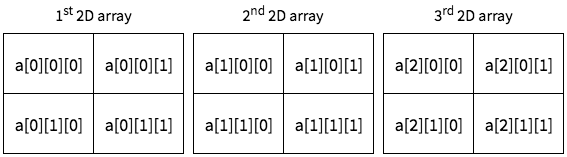To use any element of the array, you need to use three indexes. For example, writing a will access the first 2D array’s second row and second column.

You can also use initializer lists with 3D arrays. Here is an example:

int a = {
{
{10, 20},
{30, 40},
},
{
{1, 2},
{4, 5}
},
{
{3, 5},
{7, 11}
}
};


In the above example, the first 2D array’s first row is initialized to 10, 20, and the second row is initialized to 30, 40. Similarly, the second 2D array’s first row is initialized with 1, 2; the second row is initialized to 4, 5, and so on.

To iterate over a 3D array, you need to nest three for loops. The outer loop to change the outermost index. The loop inside it changes the middle index, and the innermost loop changes the last index. To see what we mean, take a look at the example below which initializes the array and prints all its elements. You can download it here.

Similarly, for a four dimensional array, you need to nest four for loops, and access it with four indexes, like a.

## Strings in C: Arrays of Characters

In C, strings are simply an array of characters followed by the “null terminating character”. The null terminating character is a character with the integer value zero, and it is used to represent the end of a string.

Let us see how you can define a string. If you want to create a string with the content “world”, you can use the “initializer string” syntax. It looks like this:

char s[] = "world";

The array s is six characters in length, because, apart from the characters “w”, “o”, “r”, “l”, “d”, there is also a null terminating character in the array.

You can also use the “initializer list” syntax to declare strings. However, in this case, the C compiler won’t put in the null terminating character for you, so you have to manually put it in. In C, '\0' is used to represent this character, so you would have to use:

char string[] = {'w', 'o', 'r', 'l', 'd', '\0'};


With the initializer list/string syntax, you can also set the array size manually if you wish to, but this isn’t necessary:

char string = {'w', 'o', 'r', 'l', 'd', '\0'};
char string = "world"; // same as above


However, there is one special case. If you write something like this:

char string = "world";

The C compiler will declare an array of five characters. However, since there is no space to fit the null terminating character, it will not add it to the string. However, for this example, you can’t put a size less than 5, and C compilers will throw an error.

char string = "world"; // wrong!

C provides multiple string manipulation functions in the header file string.h. However, this is outside the scope of arrays, so we will not discuss it here.

If you liked this post, please share it :)